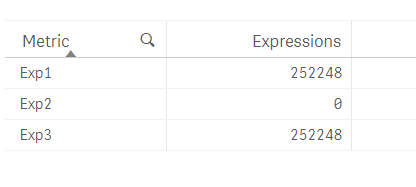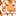Announcements
Applications are open for the 2024 Qlik Luminary Program. Apply by December 15 here.
cancel
Showing results for
Did you mean:Anonymous
Not applicable

## Aggr Expressions NOT Working when using ValueList() to create Synthetic Dimension

I have attached a sample Qlik Sense App that contains a straight table with Custom Dimensions and Expressions.

Here you would see that the second expression DOES NOT generate any output, even though it is the same expression. The only thing that stands out to me is that, the second instance of AGGR in such scenario doesn't work.

Front End TableTable Expression Code

Dimension:

=ValueList('Exp1', 'Exp2', 'Exp3')

Expression:

Pick(Match(ValueList('Exp1', 'Exp2', 'Exp3'), 'Exp1', 'Exp2', 'Exp3')

, Sum(Aggr(Sum(Expression1), Dim1))

, Sum(Aggr(Sum(Expression1), Dim1))

, Sum(Expression1)

)

Any help is appreciated.

1 Solution

Accepted SolutionsAnonymous
Not applicable
Author

Here is the solution that worked for me:

Create island tables with the Dummy Dimensions in the Data Model (instead of using ValueList() function to create the synthetic dimensions) and include the Dimension Field in grouping.

Dimension

KPI_Dimension:

KPI_Metric

Exp1

Exp2

Exp3

];

Expression:

Pick(Match(ValueList('Exp1', 'Exp2', 'Exp3'), 'Exp1', 'Exp2', 'Exp3')

, Sum(Aggr(Sum(Expression1), Dim1, KPI_Metric))

, Sum(Aggr(Sum(Expression1), Dim1, KPI_Metric))

, Sum(Expression1)

)

This solves the problem of creating unique aggregations, which is not possible using ValueList(), where the Qlik Engine doesn't understand each dummy value needs a distinct grouping.

6 RepliesMVP

Try

Pick(Match(ValueList('Exp1', 'Exp2', 'Exp3'), 'Exp1', 'Exp2', 'Exp3')

, Sum(TOTAL Aggr( Sum(Expression1), Dim1))

//, Sum(Aggr(Sum(Expression1), Dim1))

, Sum(TOTAL Aggr( Sum(Expression1), Dim1))

, Sum(Expression1)

)MVPAnonymous
Not applicable
Author

Here is the solution that worked for me:

Create island tables with the Dummy Dimensions in the Data Model (instead of using ValueList() function to create the synthetic dimensions) and include the Dimension Field in grouping.

Dimension

KPI_Dimension:

KPI_Metric

Exp1

Exp2

Exp3

];

Expression:

Pick(Match(ValueList('Exp1', 'Exp2', 'Exp3'), 'Exp1', 'Exp2', 'Exp3')

, Sum(Aggr(Sum(Expression1), Dim1, KPI_Metric))

, Sum(Aggr(Sum(Expression1), Dim1, KPI_Metric))

, Sum(Expression1)

)

This solves the problem of creating unique aggregations, which is not possible using ValueList(), where the Qlik Engine doesn't understand each dummy value needs a distinct grouping.Specialist

Any idea how to get this set analysis right

the first line works the others are zeroPICK( Match(ORDEM1,
\$(=Concat({<ORDEM1 -= {''}>}DISTINCT chr(39) & ORDEM1 & chr(39),',',ORDEM1))
),
sum({<Ano={\$(=Max(Ano))},[Mes]={'Fev'}>}Aggr(\$(vExpressao1), Ano,Mes)),sum({<Ano={\$(=Max(Ano))},[Mes]={'Fev'}>}Aggr(\$(vExpressao2), Ano,Mes)),
sum({<Ano={\$(=Max(Ano))},[Mes]={'Fev'}>}Aggr(\$(vExpressao3), Ano,Mes)),sum({<Ano={\$(=Max(Ano))},[Mes]={'Fev'}>}Aggr(\$(vExpressao4), Ano,Mes))

)

NevesSpecialist

Sunny,

the first line works the others are zero

set analysis below
NevesContributor II

Hi,

What should come in Expression1?
And where should we define it? Data load or write it as expression?

Dimension
KPI_Dimension:
KPI_Metric
GMV
Discounts
Cancellation
Return
NetSales
];

My GMV Expression : (Sum(Aggr(Only(line_items_price), [order_id]))).
Not sure how to include this in

Pick(Match(ValueList('Exp1', 'Exp2', 'Exp3'), 'Exp1', 'Exp2', 'Exp3')

, Sum(Aggr(Sum(Expression1), Dim1, KPI_Metric))

, Sum(Aggr(Sum(Expression1), Dim1, KPI_Metric))

, Sum(Expression1)

)

to make it work.Tags
Community Browser